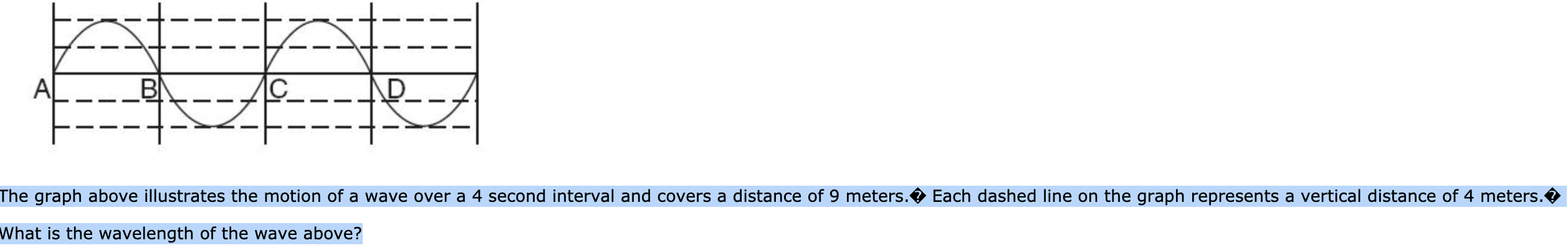# CBIAThe graph above illustrates the motion of a wave over a 4 second interval and covers a distance of 9 meters.Each dashed line on the graph represents a vertical distance of 4 meters.What is the wavelength of the wave above?

Question
1 viewshelp_outlineImage TranscriptioncloseC BI A The graph above illustrates the motion of a wave over a 4 second interval and covers a distance of 9 meters. Each dashed line on the graph represents a vertical distance of 4 meters. What is the wavelength of the wave above? fullscreen
check_circle

Step 1

Wavelength is the distance between tw...

### Want to see the full answer?

See Solution

#### Want to see this answer and more?

Solutions are written by subject experts who are available 24/7. Questions are typically answered within 1 hour.*

See Solution
*Response times may vary by subject and question.
Tagged in

### Alcohols, phenols and ether